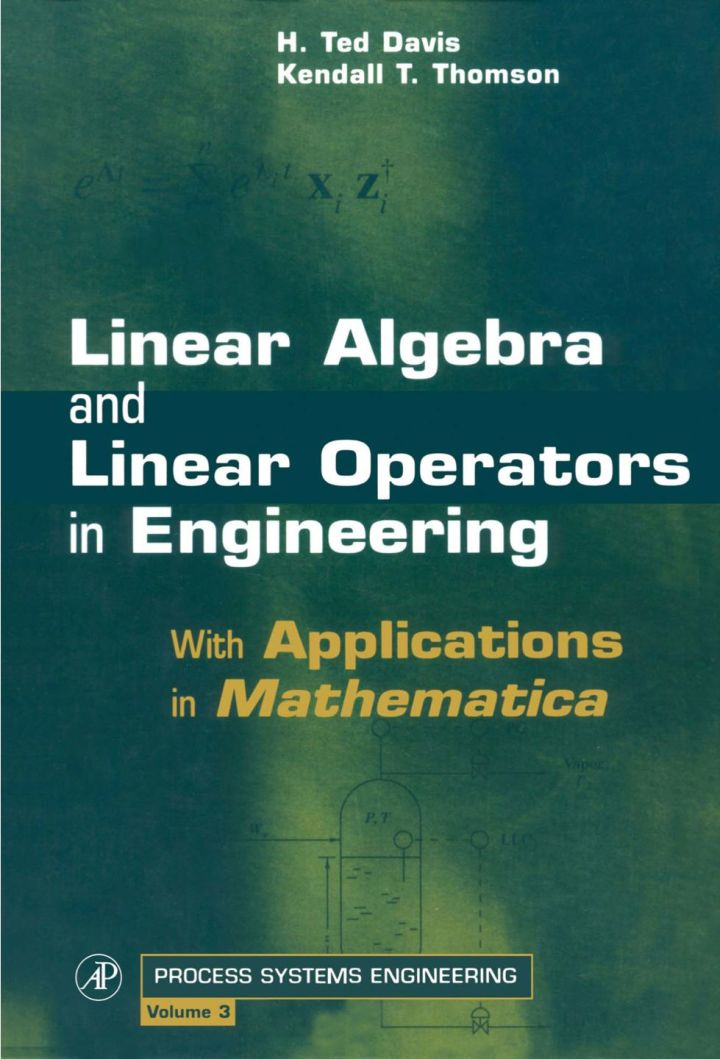# Linear Algebra and Linear Operators in Engineering: With Applications in Mathematica®

## Linear Algebra and Linear Operators in Engineering: With Applications in Mathematica®

By Davis, H. Ted; Thomson, Kendall T.

### Rent or Buy eTextbook

Expires on Apr 23rd, 2022
\$155

Publisher List Price: \$179.99
Savings: \$24.99

Designed for advanced engineering, physical science, and applied mathematics students, this innovative textbook is an introduction to both the theory and practical application of linear algebra and functional analysis. The book is self-contained, beginning with elementary principles, basic concepts, and definitions. The important theorems of the subject are covered and effective application tools are developed, working up to a thorough treatment of eigenanalysis and the spectral resolution theorem. Building on a fundamental understanding of finite vector spaces, infinite dimensional Hilbert spaces are introduced from analogy. Wherever possible, theorems and definitions from matrix theory are called upon to drive the analogy home. The result is a clear and intuitive segue to functional analysis, culminating in a practical introduction to the functional theory of integral and differential operators. Numerous examples, problems, and illustrations highlight applications from all over engineering and the physical sciences. Also included are several numerical applications, complete with Mathematica solutions and code, giving the student a "hands-on" introduction to numerical analysis. Linear Algebra and Linear Operators in Engineering is ideally suited as the main text of an introductory graduate course, and is a fine instrument for self-study or as a general reference for those applying mathematics.

· Contains numerous Mathematica examples complete with full code and solutions
· Provides complete numerical algorithms for solving linear and nonlinear problems
· Spans elementary notions to the functional theory of linear integral and differential equations
· Includes over 130 examples, illustrations, and exercises and over 220 problems ranging from basic concepts to challenging applications
· Presents real-life applications from chemical, mechanical, and electrical engineering and the physical sciences

Subject: Mathematics & Statistics -> Mathematics -> Mathematics GeneralPublisher: Elsevier S & T 07/2000
Imprint: Academic Press
Language: English
Length: 547 pages

ISBN 10: 012206349X
ISBN 13: 9780122063497
Print ISBN: 9780122063497

Live Chats